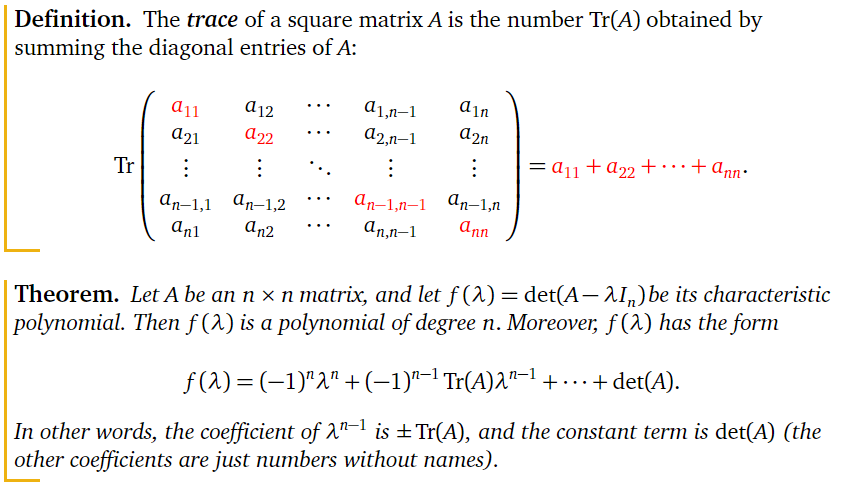## Example

I am taking a look at the following question to create a function to find the characteristic polynomial of a matrix.

Find the characteristic polynomial of the matrix:

A <- rbind(c(3,2,1), c(0,1,1), c(1,2,0))
print(A)
##      [,1] [,2] [,3]
## [1,]    3    2    1
## [2,]    0    1    1
## [3,]    1    2    0

### Theorem### Solution

The following code block prints the characteristic polynomial for a 3x3 matrix. I am interested in finding more general solutions for this problem as well.

polychar <- function(matrix){
traces = c()
i = 1
n = nrow(matrix)
while (i < (n + 1)){
traces <- c(traces, matrix[i,i])
i = i+1}
tr = sum(traces)
poly = paste0((-1)^n,'x^', n, ' + ', tr, 'x^', n-1, ' + ',det(A))
return(poly)
}

polychar(A)
##  "-1x^3 + 4x^2 + -5"